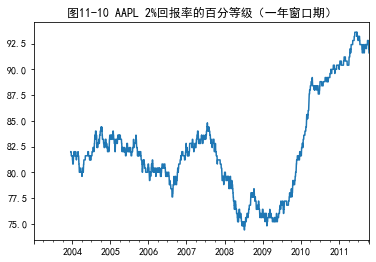signed

QiShunwang

“诚信为本、客户至上”

# 利用python进行数据分析--时间序列（下）

2021/3/21 8:10:42   来源：

### 文章目录

• 时期及其算术运算
• 时期的频率转换
• 按季度计算的时期频率
• 将Timestamp转换为Period（及其反向过程）
• 通过数组创建PeriodIndex
• 重采样及频率转换
• 降采样
• OHLC重采样
• 升采样和插值
• 通过时期进⾏重采样
• 移动窗⼝函数
• 指数加权函数
• ⼆元移动窗⼝函数
• ⽤户定义的移动窗⼝函数

## 时期及其算术运算

``````p = pd.Period(2007, freq='A-DEC')
p
``````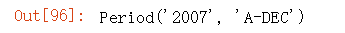``````p + 5
``````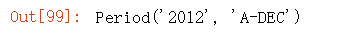``````p - 2
``````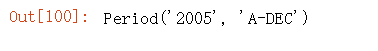``````pd.Period('2014', freq='A-DEC') - p
``````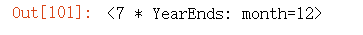period_range函数可⽤于创建规则的时期范围：

``````rng = pd.period_range('2000-01-01', '2000-06-30', freq='M')
rng
``````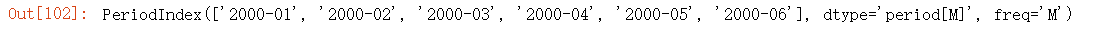PeriodIndex类保存了⼀组Period，它可以在任何pandas数据结构中被⽤作轴索引：

``````pd.Series(np.random.randn(6), index=rng)
``````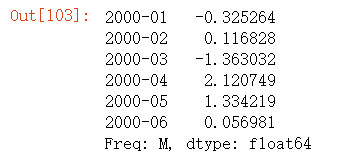``````values = ['2001Q3', '2002Q2', '2003Q1']
index = pd.PeriodIndex(values, freq='Q-DEC')
index
``````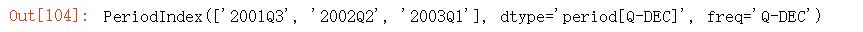### 时期的频率转换

Period和PeriodIndex对象都可以通过其asfreq⽅法被转换成别的频率。假设我们有⼀个年度时期，希望将其转换为当年年初或年末的⼀个⽉度时期。该任务⾮常简单：

``````p = pd.Period('2007', freq='A-DEC')
p
``````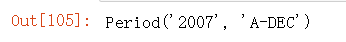``````p.asfreq('M', how='start')
``````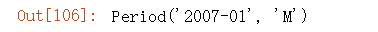``````p.asfreq('M', how='end')
``````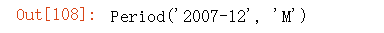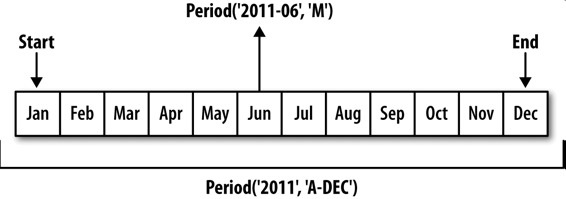``````p = pd.Period('2007', freq='A-JUN')
p
``````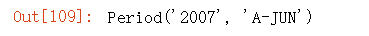``````p.asfreq('M', 'start')
``````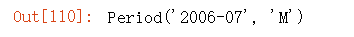``````p.asfreq('M', 'end')
``````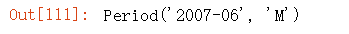``````p = pd.Period('Aug-2007', 'M')
p
``````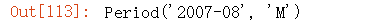``````p.asfreq('A-JUN')
``````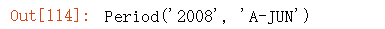``````rng = pd.period_range('2006', '2009', freq='A-DEC')
ts = pd.Series(np.random.randn(len(rng)), index=rng)
ts
``````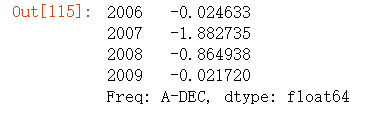``````ts.asfreq('M', how='start')
``````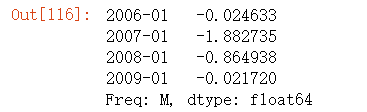``````ts.asfreq('B', how='end')
``````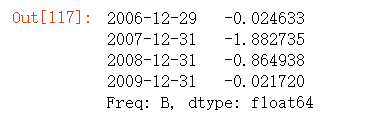### 按季度计算的时期频率

``````p = pd.Period('2012Q4', freq='Q-JAN')  # 2012Q4: 2012年第四季度
p
``````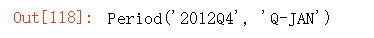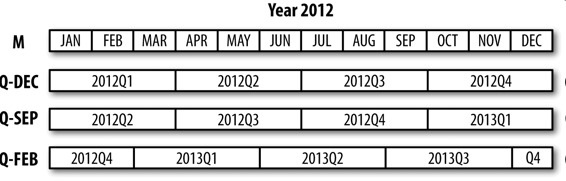``````p.asfreq('D', 'start')
``````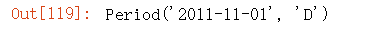``````p.asfreq('D', 'end')
``````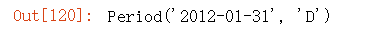``````p4pm = (p.asfreq('B', 'e') - 1).asfreq('T', 's') + 16 * 60
p4pm
``````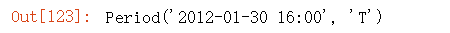``````p4pm.to_timestamp()
``````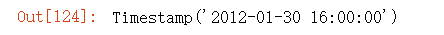period_range可⽤于⽣成季度型范围。季度型范围的算术运算也跟上⾯是⼀样的：

``````rng = pd.period_range('2011Q3', '2012Q4', freq='Q-JAN')
ts = pd.Series(np.arange(len(rng)), index=rng)
ts
``````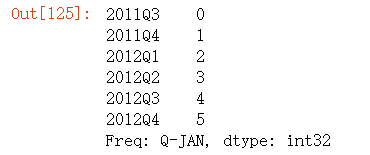``````new_rng = (rng.asfreq('B', 'e') - 1).asfreq('T', 's') + 16 * 60
ts.index = new_rng.to_timestamp()
ts
``````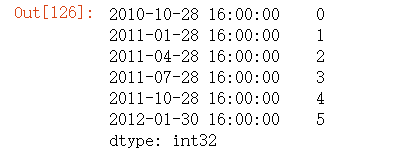### 将Timestamp转换为Period（及其反向过程）

``````rng = pd.date_range('2000-01-01', periods=3, freq='M')
ts = pd.Series(np.random.randn(3), index=rng)
ts
``````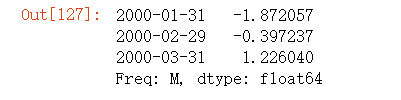``````pts = ts.to_period()
pts
``````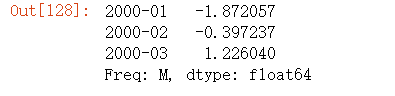``````rng = pd.date_range('1/29/2000', periods=6, freq='D')
ts2 = pd.Series(np.random.randn(6), index=rng)
ts2
``````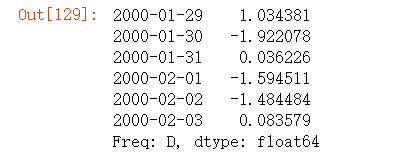``````ts2.to_period('M')
``````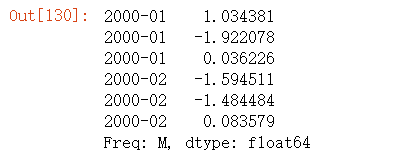``````pts = ts2.to_period()
pts
``````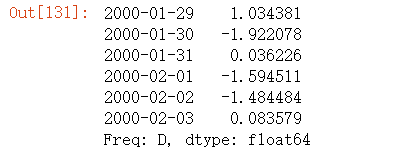``````pts.to_timestamp(how='end')
``````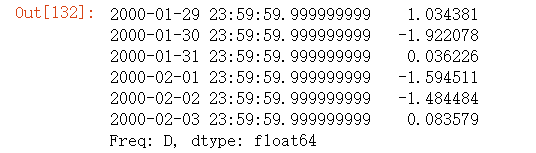### 通过数组创建PeriodIndex

``````data = pd.read_csv('./macrodata.csv')
data.head(5)
``````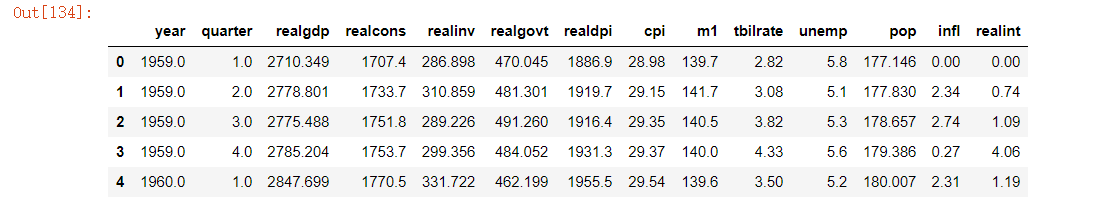``````data.year
``````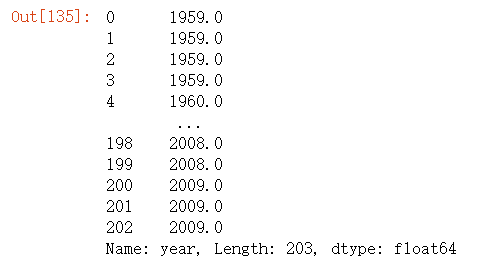``````data.quarter
``````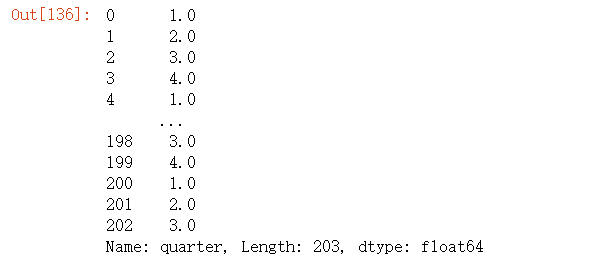``````index = pd.PeriodIndex(year=data.year, quarter=data.quarter,
freq='Q-DEC')
index
``````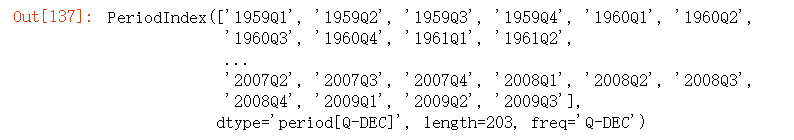``````data.index = index
data.infl
``````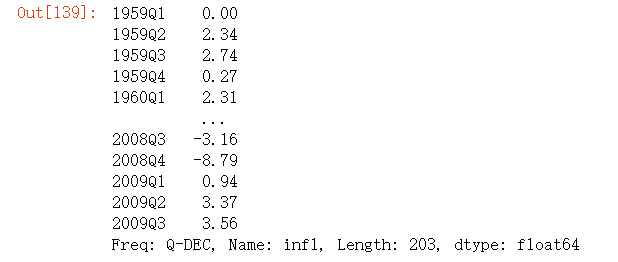## 重采样及频率转换

pandas对象都带有⼀个resample⽅法，它是各种频率转换⼯作的主⼒函数。resample有⼀个类似于groupby的API，调⽤resample可以分组数据，然后会调⽤⼀个聚合函数：

``````rng = pd.date_range('2000-01-01', periods=100, freq='D')
ts = pd.Series(np.random.randn(len(rng)), index=rng)
ts
``````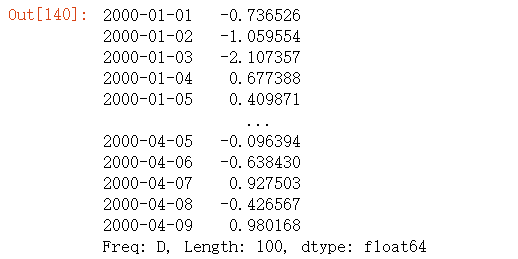``````ts.resample('M').mean()
``````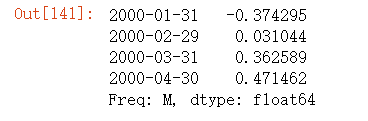``````ts.resample('M', kind='period').mean()
``````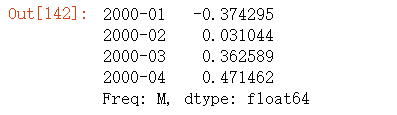resample是⼀个灵活⾼效的⽅法，可⽤于处理⾮常⼤的时间序列。我将通过⼀系列的示例说明其⽤法。表11-5总结它的⼀些选项。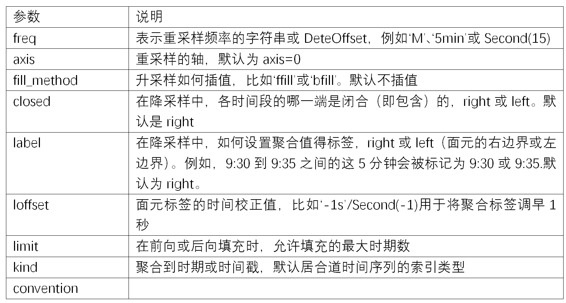### 降采样

• 各区间哪边是闭合的。
• 如何标记各个聚合⾯元，⽤区间的开头还是末尾。

``````rng = pd.date_range('2000-01-01', periods=12, freq='T')
ts = pd.Series(np.arange(12), index=rng)
ts
``````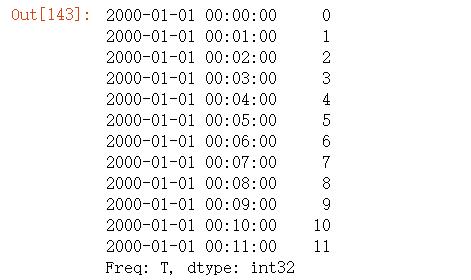``````ts.resample('5min', closed='right').sum()
``````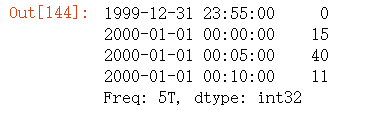``````ts.resample('5min', closed='left').sum()
``````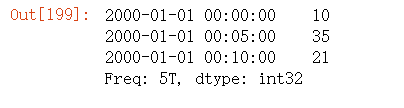``````ts.resample('5min', closed='right', label='right').sum()
``````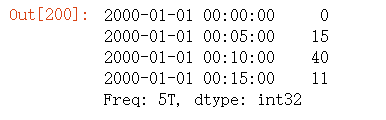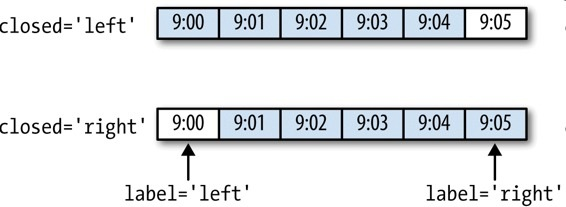``````from pandas.tseries.frequencies import to_offset
df = ts.resample("5min", closed='right',
label='right').sum()
df.index = df.index + to_offset("-1s")
df.index
``````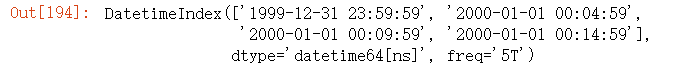``````df
``````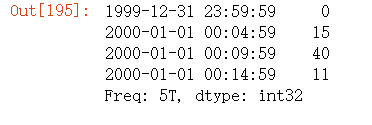### OHLC重采样

⾦融领域中有⼀种⽆所不在的时间序列聚合⽅式，即计算各⾯元的四个值：第⼀个值（open，开盘）、最后⼀个值（close，收盘）、最⼤值（high，最⾼）以及最⼩值（low，最低）。传⼊`how='ohlc'`即可得到⼀个含有这四种聚合值的DataFrame。整个过程很⾼效，只需⼀次扫描即可计算出结果：

``````ts.resample('5min').ohlc()
``````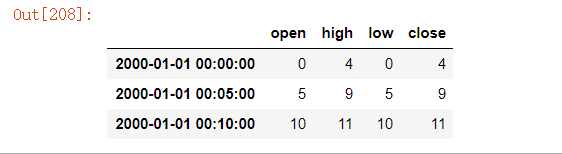### 升采样和插值

``````frame = pd.DataFrame(np.random.randn(2, 4),
index=pd.date_range('1/1/2000', periods=2,
freq='W-WED'),
columns=['Colorado', 'Texas', 'New York', 'Ohio'])
frame
``````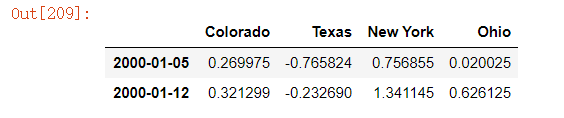``````df_daily = frame.resample('D').asfreq()
df_daily
``````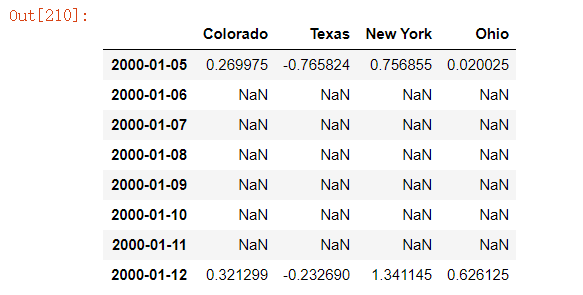``````frame.resample('D').ffill()
``````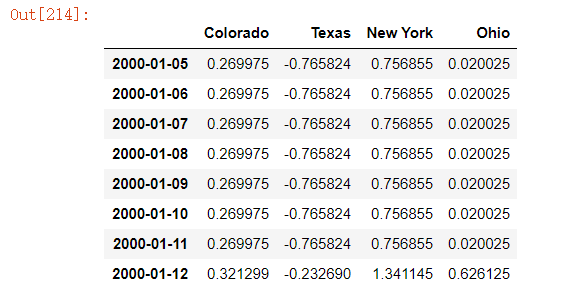``````frame.resample('D').ffill(limit=2)
``````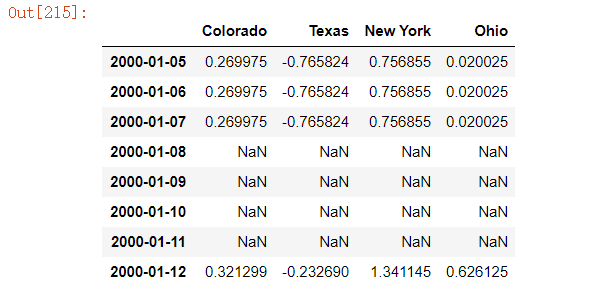``````frame.resample('W-THU').ffill()
``````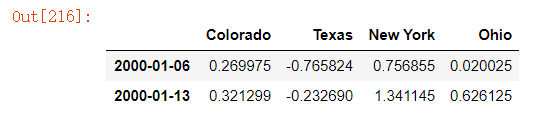### 通过时期进⾏重采样

``````frame = pd.DataFrame(np.random.randn(24, 4),
index=pd.period_range('1-2000', '12-2001',
freq='M'),
columns=['Colorado', 'Texas', 'New York', 'Ohio'])
frame[:5]
``````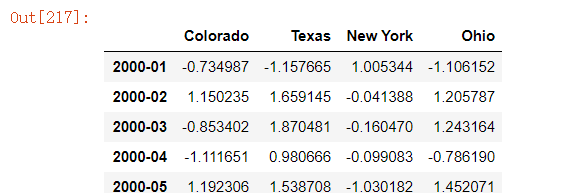``````annual_frame = frame.resample('A-DEC').mean()
annual_frame
``````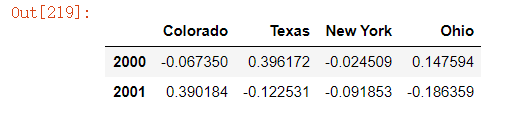``````# Q-DEC: 季度，年末12月
annual_frame.resample('Q-DEC').ffill()
``````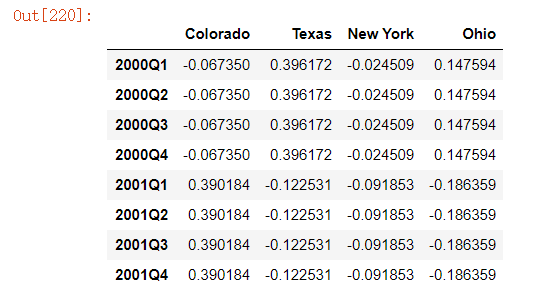``````annual_frame.resample('Q-DEC', convention='end').ffill()
``````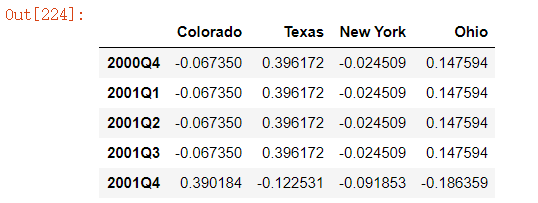• 在降采样中，⽬标频率必须是源频率的⼦时期（subperiod）。
• 在升采样中，⽬标频率必须是源频率的超时期（superperiod）。

``````annual_frame.resample('Q-MAR').ffill()
``````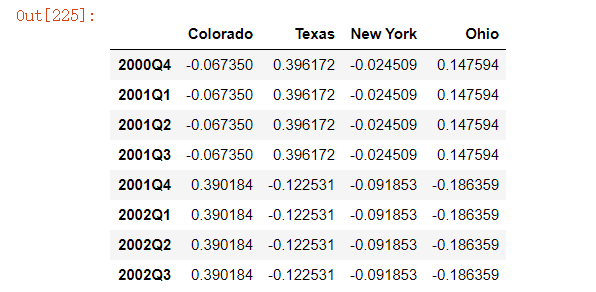## 移动窗⼝函数

``````close_px_all = pd.read_csv('./stock_px_2.csv',
parse_dates=True, index_col=0)
close_px = close_px_all[['AAPL', 'MSFT', 'XOM']]
close_px = close_px.resample('B').ffill()
close_px
``````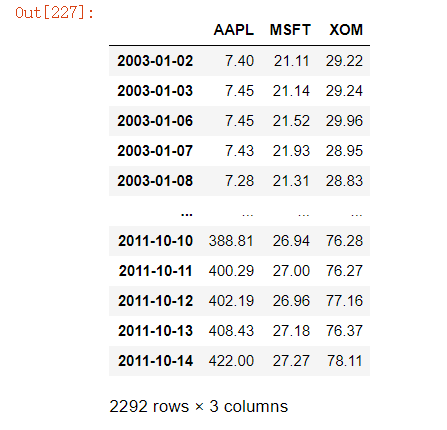``````plt.rcParams['font.sans-serif'] = ['simhei']
close_px.AAPL.plot()
close_px.AAPL.rolling(250).mean().plot().set_title('图11-4 苹果公司股价的250日均线')
``````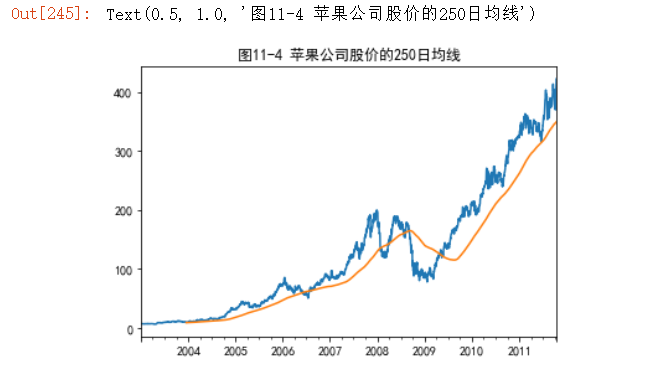``````appl_std250 = close_px.AAPL.rolling(250, min_periods=10).std()
appl_std250[5:12]
``````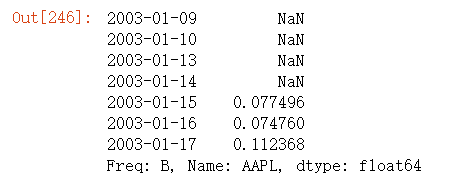``````appl_std250.plot().set_title('图11-5 苹果公司250日每日回报标准差')
``````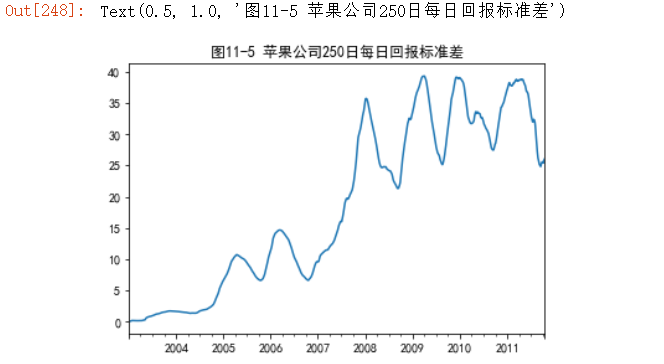``````expanding_mean = appl_std250.expanding().mean()
``````

``````close_px.rolling(60).mean().plot(logy=True).set_title('图11-6 各股价60日均线（对数Y轴）')
``````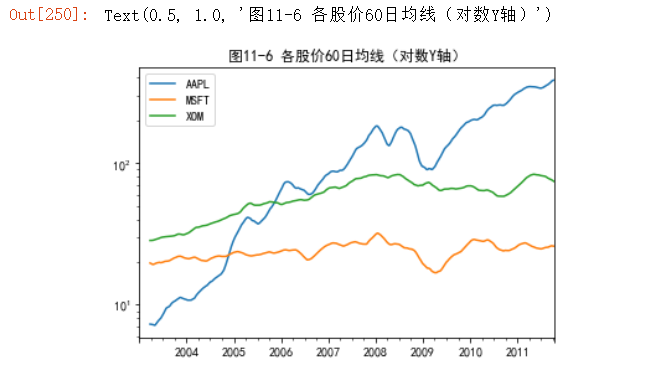rolling函数也可以接受⼀个指定固定⼤⼩时间补偿字符串，⽽不是⼀组时期。这样可以⽅便处理不规律的时间序列。这些字符串也可以传递给resample。例如，我们可以计算20天的滚动均值，如下所示：

``````close_px.rolling('20D').mean()
``````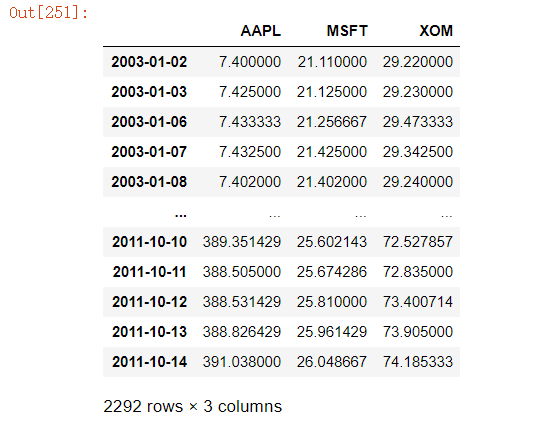### 指数加权函数

``````aapl_px = close_px.AAPL['2006':'2007']
ma60 = aapl_px.rolling(30, min_periods=20).mean()
ewma60 = aapl_px.ewm(span=30).mean()
ma60.plot(style='k--', label='Simple MA')
ewma60.plot(style='k-', label='EW MA').set_title('图11-7 简单移动平均与指数加权移动平均')
plt.legend()
``````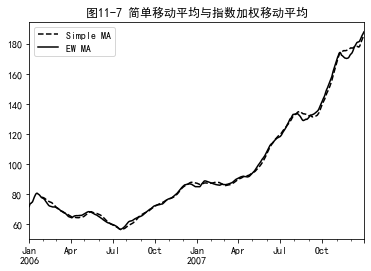### ⼆元移动窗⼝函数

``````spx_px = close_px_all['SPX']
spx_rets = spx_px.pct_change()
returns = close_px.pct_change()
``````

``````corr = returns.AAPL.rolling(125, min_periods=100).corr(spx_rets)
corr.plot().set_title('图11-8 AAPL 6个月的回报与标准普尔500指数的相关系数')
``````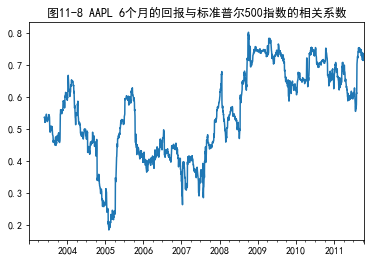``````corr = returns.rolling(125, min_periods=100).corr(spx_rets)
corr.plot().set_title('图11-9 3只股票6个月的回报与标准普尔500指数的相关系数')
``````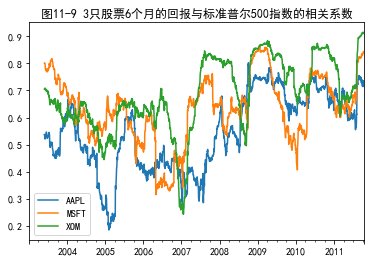### ⽤户定义的移动窗⼝函数

rolling_apply函数使你能够在移动窗⼝上应⽤⾃⼰设计的数组函数。唯⼀要求的就是：该函数要能从数组的各个⽚段中产⽣单个值（即约简）。⽐如说，当我们⽤`rolling(...).quantile(q)`计算样本分位数时，可能对样本中特定值的百分等级感兴趣。

`scipy.stats.percentileofscore`函数就能达到这个⽬的（结果⻅图11-10）：

``````from scipy.stats import percentileofscore
score_at_2percent = lambda x: percentileofscore(x, 0.02)
result = returns.AAPL.rolling(250).apply(score_at_2percent)
result.plot().set_title('图11-10 AAPL 2%回报率的百分等级（一年窗口期）')
``````